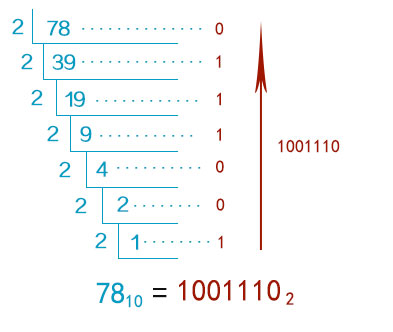# Decimal to binary

This converter can convert decimal numbers to binary numbers very quickly, both negative and floating-point numbers are supported for conversion. At the same time, you can also choose any conversion between binary to base 36. Binary to decimal

 Base(default decimal) 10(decimal) More base: 2(binary)345678(octal)910 (decimal)111213141516(Hexadecimal)1718192021222324252627282930313233343536 Decimal number Target base(default binary) 2(binary)More base: 2345678(octal)910(decimal)111213141516(Hexadecimal)1718192021222324252627282930313233343536 Conversion result

## Knowledge of converter

In mathematics and digital electronics, a binary number is a number expressed in the base-2 numeral system or binary numeral system, which uses only two symbols: typically 0 (zero) and 1 (one).

The base-2 numeral system is a positional notation with a radix of 2. Each digit is referred to as a bit. Because of its straightforward implementation in digital electronic circuitry using logic gates, the binary system is used by almost all modern computers and computer-based devices.

## How to convert decimal to binary

1. Divide the number by 2.

2. Get the integer quotient for the next iteration.

3. Get the remainder for the binary digit.

4. Repeat the steps until the quotient is equal to 0.Example

Convert 1510 to binary:

Division by 2QuotientRemainderBit #
15/2710
7/2311
3/2112
1/2013

So 1510 = 11112

## Decimal to binary conversion table

Decimal NumberBinary Number
00
11
210
311
4100
5101
6110
7111
81000
91001
101010
111011
121100
131101
141110
151111
1610000
1710001
1810010
1910011
2010100
2110101
2210110
2310111
2411000
2511001
2611010
2711011
2811100
2911101
3011110
3111111
32100000
641000000
12810000000
256100000000